### 13.2.1.1 The scalar case

To make the discussion concrete, consider the following differential equation: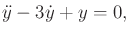(13.29)

in which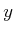is a scalar variable,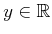. This is a second-order differential equation because of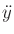. A phase space can be defined as follows. Let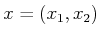denote a two-dimensional phase vector, which is defined by assigning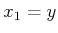and. The terms state space and state vector will be used interchangeably with phase space and phase vector, respectively, in contexts in which the phase space is defined. Substituting the equations into (13.29) yields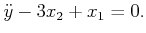(13.30)

So far, this does not seem to have helped. However,can be expressed as either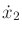or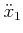. The first choice is better because it is a lower order derivative. Using, the differential equation becomes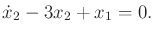(13.31)

Is this expression equivalent to (13.29)? By itself it is not. There is one more constraint,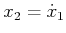. In implicit form,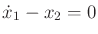. The key to making the phase space approach work correctly is to relate some of the phase variables by derivatives.

Using the phase space, we just converted the second-order differential equation (13.29) into two first-order differential equations,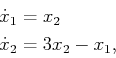(13.32)

which are obtained by solving for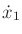and. Note that (13.32) can be expressed as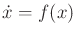, in which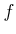is a function that maps from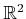into.

The same approach can be used for any differential equation in implicit form,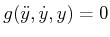. Let,, and. This results in the implicit equations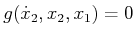and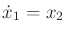. Now suppose that there is a scalar action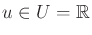represented in the differential equations. Once again, the same approach applies. In implicit form,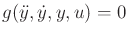can be expressed as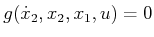.

Suppose that a given acceleration constraint is expressed in parametric form as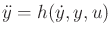. This often occurs in the dynamics models of Section 13.3. This can be converted into a phase transition equation or state transition equation of the form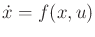, in which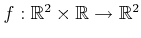. The expression is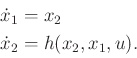(13.33)

For a second-order differential equation, two initial conditions are usually given. The values of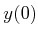andare needed to determine the exact position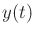for any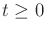. Using the phase space representation, no higher order initial conditions are needed because any point in phase space indicates bothand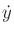. Thus, given an initial point in the phase and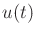for all,can be determined.

Example 13..3 (Double Integrator)   The double integrator is a simple yet important example that nicely illustrates the phase space. Suppose that a second-order differential equation is given as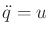, in which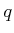andare chosen from. In words, this means that the action directly specifies acceleration. Integrating13.5 once yields the velocity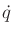and performing a double integration yields the position. If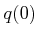and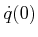are given, and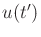is specified for all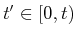, then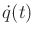and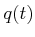can be determined for any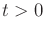.

A two-dimensional phase space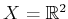is defined in which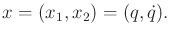(13.34)

The state (or phase) transition equationis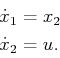(13.35)

To determine the state trajectory, initial values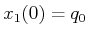(position) and(velocity) must be given in addition to the action history. Ifis constant, then the state trajectory is quadratic because it is obtained by two integrations of a constant function.Steven M LaValle 2020-08-14